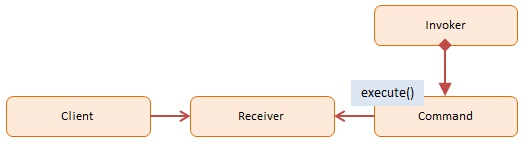# Command Design Pattern

Structural: Command Pattern

### Can you explain the command design pattern?​

Interview Response: Command Pattern is a behavioral JS design pattern that aims to encapsulate actions or operations as objects. This pattern is helpful in scenarios where we want to decouple or split the objects executing the commands from the objects issuing the orders. You may centralize the processing of these actions/operations by using command objects.

Code Example #1:The command pattern involves four participants: command, receiver, invoker, and client.

• Command – A command object knows the receiver and calls the receiver's method. The command contains values for the receiver method's arguments.
• Client – The client is responsible for creating the command object and passing it to the invoker.
• Invoker – The invoker receives the command object from the client and is exclusively responsible for calling (or invoking) a command.

``let calculator = {  add: function (x, y) {    return x + y;  },  subtract: function (x, y) {    return x - y;  },  divide: function (x, y) {    return x / y;  },  multiply: function (x, y) {    return x * y;  },};let manager = {  execute: function (name, args) {    if (name in calculator) {      return calculator[name].apply(calculator, [].slice.call(arguments, 1));    }    return false;  },};console.log(manager.execute('add', 5, 2)); // prints 7console.log(manager.execute('multiply', 2, 4)); // prints 8``

Code Example #2:This pattern's objects are as follows:

Client -- example code: the run() function

• refers to the Receiver object.

• understands how to carry out the command's associated operation
• keeps a history of commands executed (optionally)

Command -- example code: Command

• keeps track of information concerning the activity that gets taken

Invoker -- example code: the user activating the buttons.

• requests that the request carries out

``function add(x, y) {  return x + y;}function sub(x, y) {  return x - y;}function mul(x, y) {  return x * y;}function div(x, y) {  return x / y;}let Command = function (execute, undo, value) {  this.execute = execute;  this.undo = undo;  this.value = value;};let AddCommand = function (value) {  return new Command(add, sub, value);};let SubCommand = function (value) {  return new Command(sub, add, value);};let MulCommand = function (value) {  return new Command(mul, div, value);};let DivCommand = function (value) {  return new Command(div, mul, value);};let Calculator = function () {  let current = 0;  let commands = [];  function action(command) {    let name = command.execute.toString().substr(9, 3);    return name.charAt(0).toUpperCase() + name.slice(1);  }  return {    execute: function (command) {      current = command.execute(current, command.value);      commands.push(command);      console.log(action(command) + ': ' + command.value);    },    undo: function () {      let command = commands.pop();      current = command.undo(current, command.value);      console.log('Undo ' + action(command) + ': ' + command.value);    },    getCurrentValue: function () {      return current;    },  };};function run() {  let calculator = new Calculator();  // issue commands  calculator.execute(new AddCommand(100));  calculator.execute(new SubCommand(24));  calculator.execute(new MulCommand(6));  calculator.execute(new DivCommand(2));  // reverse last two commands  calculator.undo();  calculator.undo();  console.log('\nValue: ' + calculator.getCurrentValue());}run();/*OUTPUT:Add: 100Sub: 24Mul: 6Div: 2Undo Div: 2Undo Mul: 6Value: 76*/``

### To which pattern family does the Command pattern belong?​

Interview Response: The Command pattern is a type of behavioral design pattern.

### When should you utilize the JavaScript command pattern?​

Interview Response: Use Case:

• If you want to queue and execute requests at different times.
• If you're going to perform operations such as reset or undo.
• If you're going to keep a history of requests made.